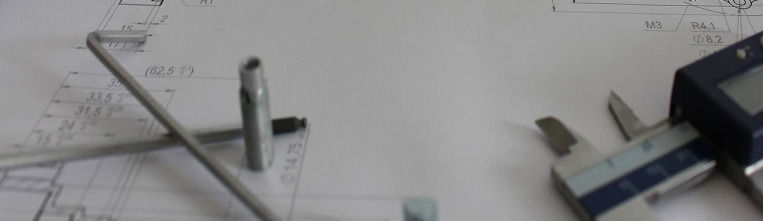Accelerometer Design

# Accelerometer Design

Now we know enough to understand  the basic function of an accelerometer which we want to look at in this chapter.  We also will see some design considerations which are dictated by the environmental constraints and then the basic set-ups using the different working modes of the piezo-element. Finally we will have a look at the electric output of the sensor with a short overview of common practice electronics used.

### Functional Principle

We retain:

• The piezoelectric accelerometer can only measure dynamic acceleration.
Below a certain minimal frequency the output becomes smaller and smaller.

• At static conditions the output becomes zero.

• The sensitivity is proportional to the mass of the inertial mass and the piezoelectric constant of the sensing element.

## Basic Principles

### Two Fundamental Properties

#### Accelerometer Output

The charge output of

the accelerometer is

​q  = d33 ·m·a

m   = mass
a    = acceleration

d33 = piezoelectric constant

(in compression mode)That means the charge signal is a true picture of the acceleration acting on the sensor. So if the mechanical input is a sinusoidal motion the output will be a sine as well.

If you need also the plus or minus sign of the signal you have to remember that the acceleration is opposite to the displacement.

The sensitivity is only depending on the piezoelectric constant and the mass. All technical materials show a certain variation of their characteristics and so does the piezoelectric constant. In practice we see often up to 10% variation. If we want the final sensitivity to be in a closer tolerance than that, we can adapt the mass accordingly. This is why the mass is designed to be about 10 to 15% heavier than really required so all sensitivities come out high. The final sensing element can then be calibrated by removing weight from the mass.

#### Discharge Curve

The charge q on a piezo element will slowly decay to zero when it is left on it’s own. This is due to a very small leakage current  between the plus side and the minus side of the piezo element. As a result the charges on both sides equal out after a while.

The duration of this relaxation is governed by the so called time constant which in turn depends on the electrical resistance between the electrodes and the capacity of the piezo-element.charge

time

Natural discharge curve

The time constant is an important characteristic of the accelerometer. We have seen that the vibration must be “quicker” than the relaxation of the signal.

The piezoelectric accelerometer is not able to measure static (continuous) acceleration. The minimal frequency that can be measured is depending on the time constant of the piezo element

### Functional Modes

We find typically two function modes or principles used with the classic piezo accelerometers i.e. the compression-mode and shear-mode.

#### Compression Mode

The figure shows a compression-mode accelerometer.

Let’s define the parts between the base and the inertial mass as the sensing element.

The sensing element in this case consists of two insulation rings, two electrodes with wires and finally the piezo element. The parts are normally held together by a central bolt but they might as well be glued or soldered.

The compression mode accelerometer is probably the most common design as it is most simple and has very good basic characteristics.Compression-mode accelerometer

#### Shear Mode

Another most popular design is the shear-mode accelerometer. The variant shown on the right is a symmetric design with two identical sensing elements. The base part includes an central support plate. Two identical sensing elements are mounted left and right with the respective inertial weights at the ends.

A characteristic of the shear-mode is that the piezo elements are upright (in the direction of the sensitivity) with the electrodes side-wards.

Sometimes only one half of the set-up is used which results in a more simple design with only one sensing element.Shear-mode accelerometer

#### Stacked Sensing-Element

In order to increase the sensitivity we can use several piezo elements on top of each other in a stack. By using for example 3 piezo rings instead of one we will get 3 times the sensitivity. All the plus poles are connected together and all the minus poles are connected together in parallel to one output.

The use of stacks is possible in compression and shear mode designs.Compression-mode accelerometer with multiple elements

#### Non-Insulated Designs

The three examples above are all fully insulated or floating. However there are also designs where the sensing element is not insulated with respect to the housing which simplifies the design considerably.

A popular shear-mode design variant is using a tube shaped piezo element and inertial mass mounted around a center post. This results in a very compact and rigid design. The parts are normally soldered together. The base (together with the housing) is then one electrical pole of the sensing element while the inertial mass the other.

This kind of electrical layout is also called single ended as it has only one live pole, the other pole being the housing.Accelerometer with shear tube element